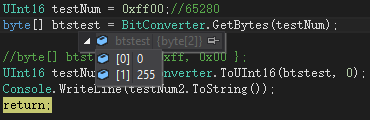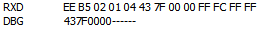• 2022-11-09
• 浏览 (392)

# 首先是字节序说明和测试:

little字节序，即小端字节序 高字节数据在地址或者数组的高位 ，低字节数据在地址或者数组的低位。这也是符合我们正常人思维理解概念的一种字节序 ，且也是大多数编程环境支持的一种默认处理方式。

c#中默认是intel架构 的little字节序 所有的字节数据处理也是遵循的。好的我们 首先编写一段简单的不能再简单的代码

``````1 UInt16 testNum = 0xff00;//65280
2 byte[] btstest = BitConverter.GetBytes(testNum);
3
4 //byte[] btstest2 = { 0xff, 0x00 };
5 UInt16 testNum2 = BitConverter.ToUInt16(btstest, 0);
6 Console.WriteLine(testNum2.ToString());
``````# 另外一种处理方式的来由

`````` 1 float oxMeasure = float.Parse(textBox1.Text);
2 //0,0,127,67
3 //0x43 7f 00 00
4 //{0x00,0x00 ,0x7f,0x43}
5 byte[] oxmeasureData = BitConverter.GetBytes(oxMeasure);
6
7 byte[] uploadData = { 0xEE, 0xB5, 0x02, 0x01, 0x04, 0x01, 0x01, 0x01, 0x01, 0xFF, 0xFC, 0xFF, 0xFF };
8 //高低位反向
``````

`````` 1 function measureProcess(parid, parData)
2     local measureHexStr = "";
3     local measureval = 0.0;
4     for i = 1, #parData, 1 do
5         measureHexStr = measureHexStr .. string.format('%02X', parData[i]);
6     end
7     --print(measureHexStr.."------");
8     measureval = hexToFloat(measureHexStr);
9     if parid == 1 then --通道1
10         globalvar_ch1_measure = measureval;
11     elseif parid == 2 then --通道2
12         globalvar_ch2_measure = measureval;
13     elseif parid == 3 then
14         globalvar_ch3_measure = measureval;
15     elseif parid == 4 then
16         globalvar_ch4_measure = measureval;
17     end
18
19 end
```````````` 1 function hexToFloat( hexString )
2     if hexString == nil then
3         return 0
4     end
5     local t = type( hexString )
6     if t == "string" then
7         hexString = tonumber(hexString , 16)
8     end
9
10     local hexNums = hexString
11
12     local sign = math.modf(hexNums/(2^31))
13
14     local exponent = hexNums % (2^31)
15     exponent = math.modf(exponent/(2^23)) -127
16
17     local mantissa = hexNums % (2^23)
18
19     for i=1,23 do
20         mantissa = mantissa / 2
21     end
22     mantissa = 1+mantissa
23 --    print(mantissa)
24     local result = (-1)^sign * mantissa * 2^exponent
25     --保留一位小数
26     result = math.floor(result * 10+ 0.5) / 10;
27     return result
28 end
``````

0